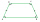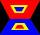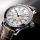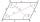Isosceles trapezium

Trapezoid YSED (YS||ED) is isosceles.
The size of the angle at vertex Y is 17 degrees.
Calculate the size of the angle at vertex E.

Result

angle DES:  163 °

Solution:Leave us a comment of example and its solution (i.e. if it is still somewhat unclear...):Be the first to comment!Next similar examples:

1. Isosceles trapezoid v3In an isosceles trapezoid ABCD is the size of the angle β = 81° Determine size of angles α, γ and δ.
2. Rectangular trapezoidHow many inner right angles has a rectangular trapezoid?
3. Minute angleDetermine size of angle, which takes minute hand for 75 minutes.
4. Angles 1It is true neighboring angles have not common arm?
5. Rhombus anglesIf one angle in the rhombus is 159°, what is it neighboring angle in rhombus?
6. Obtuse angleWhich obtuse angle is creating clocks at 17:00?
7. ParallelogramIn the parallelogram we know one internal angle 67°33`. Calculate the other internal angles.
8. Fraction to decimalWrite the fraction 3/22 as a decimal.
9. Write decimalsWrite in the decimal system the short and advanced form of these numbers: a) four thousand seventy-nine b) five hundred and one thousand six hundred and ten c) nine million twenty-six
10. Lengths of the poolMiguel swam 6 lengths of the pool. Mat swam 3 times as far as Miguel. Lionel swam 1/3 as far as Miguel. How many lengths did mat swim?
11. ZdeněkZdeněk picked up 15 l of water from a 100-liter full-water barrel. Write a fraction of what part of Zdeněk's water he picked.
12. LineCan we construct a line segment, if we know: center
13. Fraction and a decimalWrite as a fraction and a decimal. One and two plus three and five hundredths
14. Bus 14Boatesville is 65.35 kilometers from Stanton. A bus traveling from Stanton is 24.13 kilometers from Boatesville. How far has the bus traveled?Added together and write as decimal number: LXVII + MLXIVWrite the mixed number as an improper fraction. 166 2/3Simplify the following problem and express as a decimal: 5.68-[5-(2.69+5.65-3.89) /0.5]